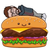# New to Qlik Sense

If you’re new to Qlik Sense, start with this Discussion Board and get up-to-speed quickly.

Announcements
JAN. 24, Q&A with Qlik: Making the Move to Qlik Cloud Reporting: REGISTER NOW
cancel
Showing results for
Did you mean:Contributor III

## Nested aggregation / calculation

Hi,

I have a calculated value in my chart: ((Sum(A)-Sum(B))/Sum(C)*100))

Now i want to add different colums to a table where with a condition a Field X

SO: {<FieldX=10>} ((Sum(A)-Sum(B))/Sum(C)*100))

I want it to be a fixed column, so regardless of the made selections.

can anyone help?

Thx

1 Solution

Accepted SolutionsCreator III

(Sum( {1<FieldX={'10'}>} A)-Sum({1<FieldX={'10'}>}B))/Sum({1<FieldX={'10'}>}C)*100)